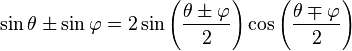# A double-slit (Young experiment) problem

## Homework Statement

In Young's double-slit interference experiment with light of wavelength $$\lambda$$, two light waves come from the slits and are superposed at the point P on the screen. These waves have the following forms:

E1 = E0 sin(wt + π/4)
E2 = E0 sin(wt + Φ)

Find the possible values of Φ if we have destructive interference at the point P.

## Homework Equations## The Attempt at a Solution

I attempted the problem two different ways and I had two different results.

Φ = 2kπ - π/4
Φ = 2kπ + 5π/4

Can you show us how you got that?

Phase difference is Φ - π/4.

So d/λ = (Φ - π/4)/2π

d = (2k+1)/2 λ for destructive interference

So, (2k+1)/2 λ = (Φ - π/4)/2π λ

Φ = 2kπ + 5π/4

-------------

The second way, I added 2 wave functions together and I equated the expression to zero.

I found Φ = 2kπ - π/4

How did you arrive at Φ = 2kπ - π/4?

Let's see. The sum of the two waves is:

E = 2E0 sin(wt + π/8 + Φ/2) cos(π/8 - Φ/2) = 0

That is satisfied when

wt + π/8 + Φ/2 = kπ

wt=2πft=2π, so we can drop it.

Φ = 2kπ - π/4

Let's see. The sum of the two waves is:

E = 2E0 sin(wt + π/8 + Φ/2) cos(π/8 - Φ/2) = 0

That is satisfied when

wt + π/8 + Φ/2 = kπ

wt=2πft=2π, so we can drop it.

Φ = 2kπ - π/4

The condition E=0 must be satisfied at ALL TIME for destructive pattern, and the bold line is not true at all time.
Find another conditionHmm, thanks hikaru. How can I proceed? I have no idea.

Because of this: "The condition E=0 must be satisfied at ALL TIME for destructive pattern"